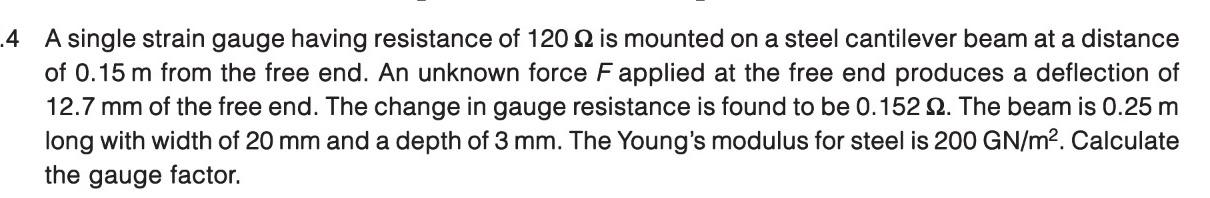# A single strain gauge having resistance of 120 Ω is mounted on a steel cantilever beam at a distance a of 0.15 m from the free end. An unknown force F applied at the free end produces a deflection of 12.7 mm of the free end. The change in gauge resistance is found to be 0.152 Ω. The beam is 0.25 m long with width of 20 mm and a depth of 3 mm. The Young’s modulus for steel is 200 GN/m2. Calculate the gauge factor.

Question-AnswerCategory: Measurement & InsturmentationA single strain gauge having resistance of 120 Ω is mounted on a steel cantilever beam at a distance a of 0.15 m from the free end. An unknown force F applied at the free end produces a deflection of 12.7 mm of the free end. The change in gauge resistance is found to be 0.152 Ω. The beam is 0.25 m long with width of 20 mm and a depth of 3 mm. The Young’s modulus for steel is 200 GN/m2. Calculate the gauge factor.

### A single strain gauge having resistance of 120 Ω is mounted on a steel cantilever beam at a distance a of 0.15 m from the free end. An unknown force F applied at the free end produces a deflection of 12.7 mm of the free end. The change in gauge resistance is found to be 0.152 Ω. The beam is 0.25 m long with width of 20 mm and a depth of 3 mm. The Young’s modulus for steel is 200 GN/m2. Calculate the gauge factor.